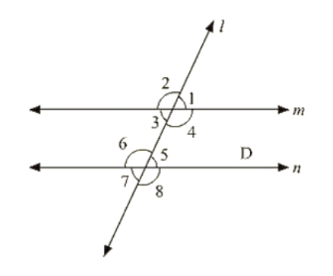# In the below fig. transversal t intersects two lines m and n, ∠4 = 110° and ∠7 = 65° is m ∥ n?

Question:

In the below fig. transversal t intersects two lines m and n, ∠4 = 110° and ∠7 = 65° is m ∥ n?Solution:

Given:

∠4 = 110° and ∠7 = 65°

To find: is m ∥ n

Here ∠7 = ∠5 = 65°       [Vertically opposite angle]

Now. ∠4 + ∠5 = 110 + 65 = 175°

Therefore, m is not parallel to n as the pair of co interior angles is not supplementary.